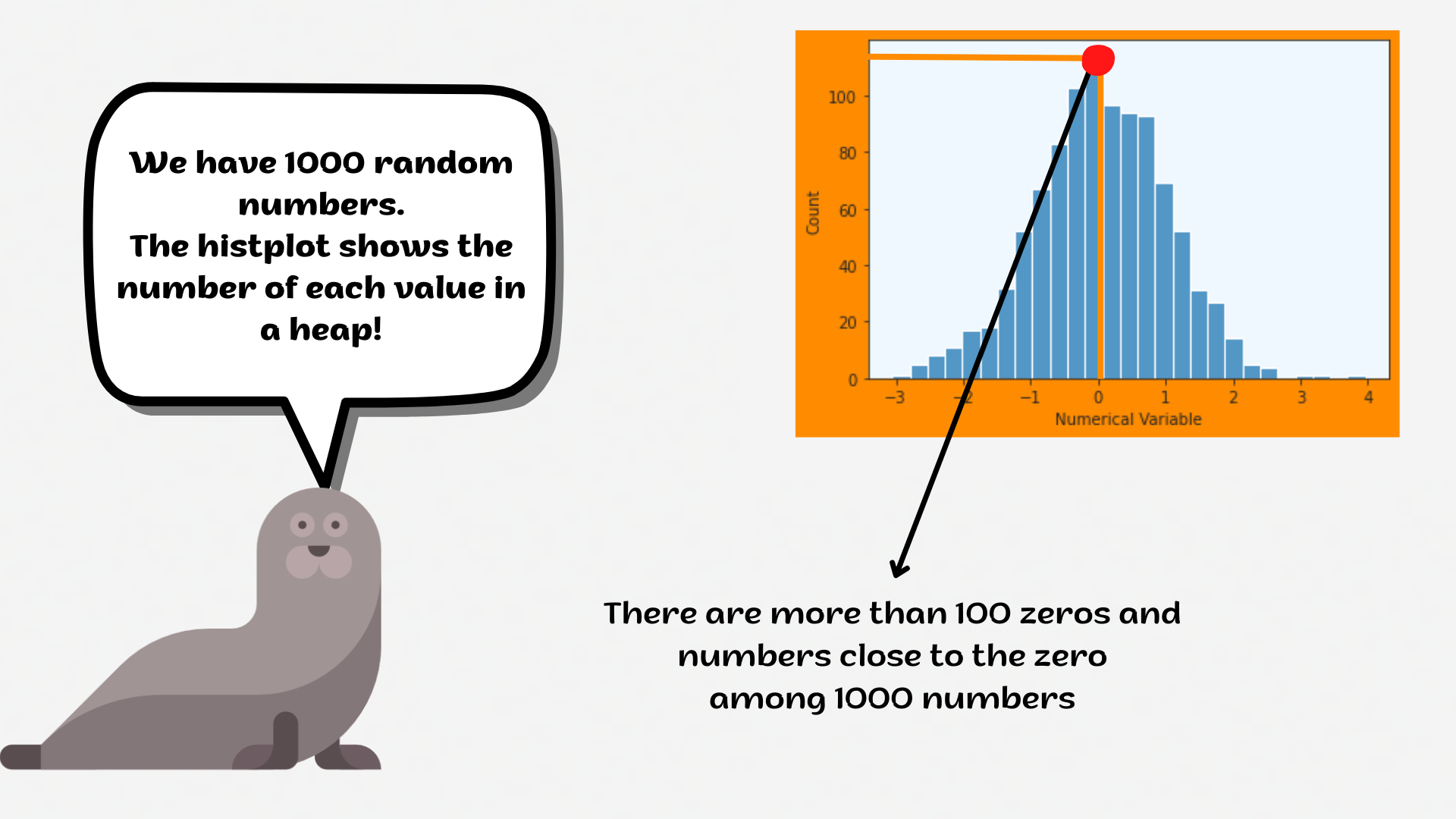Course Content

# Deep Dive into the seaborn Visualization

1. Light Start

2. Distributions of Data

3. Categorical Plot Types

4. Matrix Plots

5. Multi-Plot Grids

6. Regression Models

Deep Dive into the seaborn Visualization

##Histplot

The distributions module contains several functions designed to answer questions such as these. The axes-level functions are `histplot`, `kdeplot`, `ecdfplot`, and `rugplot`. They are grouped together within the figure-level `displot` function.A `histplot` is a classic visualization tool that represents the distribution of one or more variables by counting the number of observations that fall within discrete bins.

Click the slider to view possible arguments for the plot!Don't forget to return back after exploring the dataset!

Note

Use `plt.show()` to display the plot.

1. Create the histplot using the `seaborn` library:
• Set the `x` parameter equals the `'bill_length_mm'`;
• Set the `hue` parameter equals the `'island'`;
• Set the `element` parameter equals the `'step'`;
• Set the `stat` parameter equals the `'density'`;
• Set the `binwidth` parameter equals `1`;
• Set the `'flare'` palette;
• Use the `df` data for the plot;
• Display the plot.

Everything was clear?

Section 2. Chapter 1